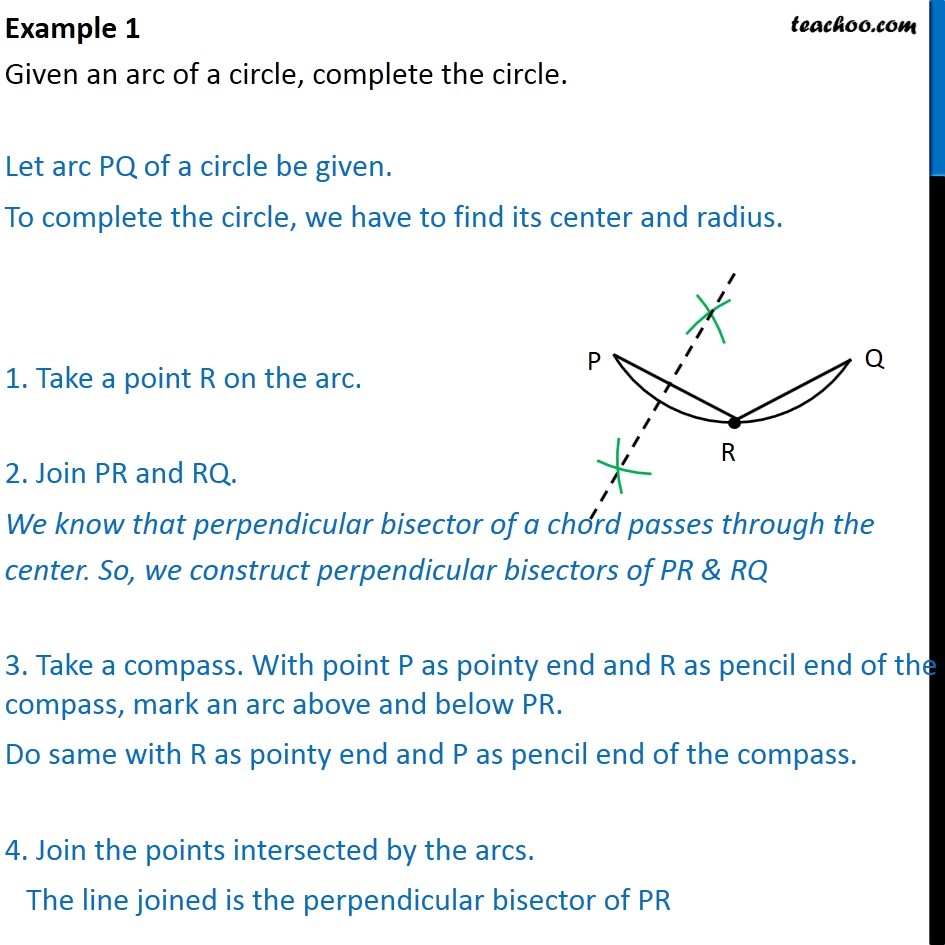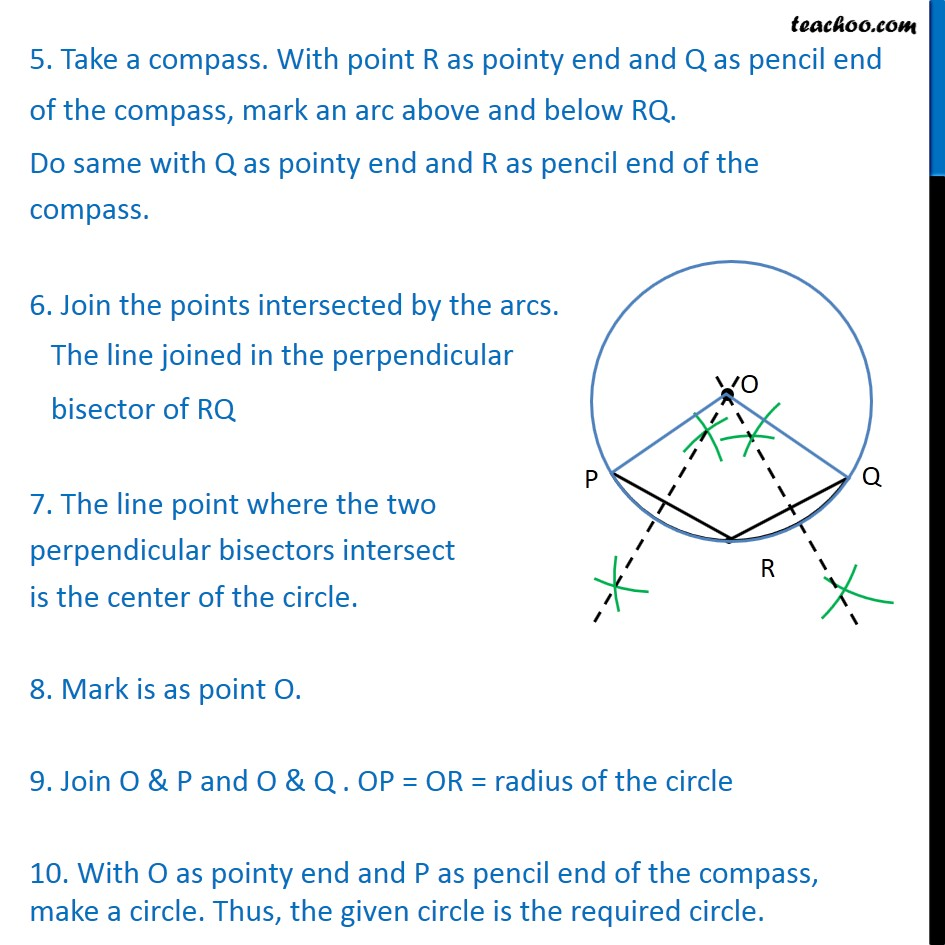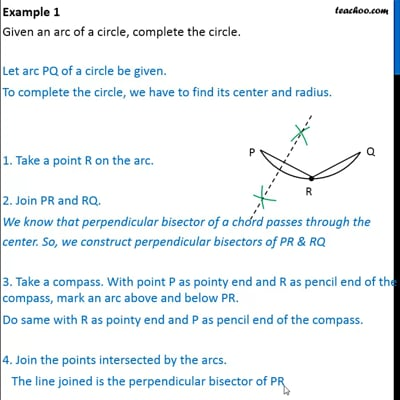Examples

Chapter 10 Class 9 Circles
Serial order wiseThis video is only available for Teachoo black users

Solve all your doubts with Teachoo Black (new monthly pack available now!)

### Transcript

Example 1 Given an arc of a circle, complete the circle. Let arc PQ of a circle be given. To complete the circle, we have to find its center and radius. 1. Take a point R on the arc. 2. Join PR and RQ. We know that perpendicular bisector of a chord passes through the center. So, we construct perpendicular bisectors of PR & RQ 3. Take a compass. With point P as pointy end and R as pencil end of the compass, mark an arc above and below PR. Do same with R as pointy end and P as pencil end of the compass. 4. Join the points intersected by the arcs. The line joined is the perpendicular bisector of PR 5. Take a compass. With point R as pointy end and Q as pencil end of the compass, mark an arc above and below RQ. Do same with Q as pointy end and R as pencil end of the compass. 6. Join the points intersected by the arcs. The line joined in the perpendicular bisector of RQ 7. The line point where the two perpendicular bisectors intersect is the center of the circle. 8. Mark is as point O. 9. Join O & P and O & Q . OP = OR = radius of the circle 10. With O as pointy end and P as pencil end of the compass, make a circle. Thus, the given circle is the required circle.